## Percentage For SBI PO : Set – 05

1) If the cost price of 20 books is the same as selling price of 25 books, then the loss percentage is

a) 20

b) 25

c) 22

d) 24

e) None of these

a)

C . P of 20 books = S.P of 25 books

20-x/x=25/100

80-4x=x

5x=80

X=16

2) The number of students in a class is increased by 20% and the number now becomes 66. Initially the number was

a) 45

b) 50

c) 55

d) 60

e) None of these

c)

Let x be number of students present initially.

Now the number of students in the class is 66

Therefore,  (120/100)*x=66

x= 55 students

3) Due to an increase of 50% in the price of eggs, 4 eggs less are available for Rs. 24.The present rate of eggs per dozen is

a) Rs. 24

b) Rs. 27

c) Rs. 36

d) Rs. 42

e) None of these

c)

Let original price of egg Rs. , then  the  increased price will be

Let original price of egg Rs. , then  the  increased price will be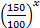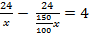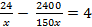3600 – 2400 = 600

= 2

Present price per dozen will be

Rs.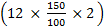= Rs. 36

3600 – 2400 = 600

= 2

4) Pradeep spends 40 percent of his monthly income on food items and 50 percent of the remaining on clothes and conveyance. He saves one-third of the remaining amount after spending on food, clothes and conveyance.  If he saves Rs.19,200 every year what is his monthly income?

a) Rs.24000

b) Rs.12000

c) Rs.16000

d) Rs.20000

e) None of these

C)

Explanation

Pradeep savings per month = 19,200/12=1600

Remaining after food, clothes & conveyance = 1600 × 3 = 4800

Monthly income = 4800/50*100*1/60*100

= 160000

5)  A man spends one third of his income on food 2/5th , of his income on house rent and 1/5th  of his income on transport. If he still has Rs.500 left with him, his income is,

a) 5000

b) 5500

c) 7500

d) 9000

e) None of these

c)

Let his income be

x-(x/3+2x/5+x/5)=500

x-14x/15=500

x/15=500

x=7500

6) The population of a city is increased 5% ,7% and 11% in the last three years, What will be the present population if the population of a town is 2,40,000 three years ago ?

a) 2,99,600

b) 2,99,500

c) 2,99,400

d) 2,99,300

e) None of these

d)

240000* 105/100*107/100*111/100 = 299300

7) 500 pencils were distributed equally among children in such a way that the number of pencil received by each child is 20% of the total number of children. How many pencil did each child receive ?

a) 45

b) 50

c) 55

d) 43

e) None of these

b)

Total no.of.children be x.

X×20/100 of X=500;

X2 =500*5

X = 50;

8) What is the maximum percentage discount that a merchant can offer so that he ends up with no profit or no loss after he marks the price 50% above the cost price?

a) 66.66%

b) 33.33 %

c) 50%

d) 40%

e) None of these

b)

Let CP = Rs. 100 and MP = Rs. 150

Rs. 50 has to be reduced so that there is no profit or no loss.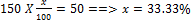9) Three candidates contested an election and received 1136, 7636 and 11628 votes respectively. What percentage of the total votes did the winning candidate get?

a) 57%

b) 60%

c) 65%

d) 90%

e) None of these

Total number of votes = 20400

Winning candidates vote = 11628

Percentage of winning candidate’s vote in total = (11628/20400)*100 = 57%

10) If 50% of x equals the sum of y and 20, then what is the value of x–2y?

a) 20

b) 40

c) 60

d) 80

e) None of these